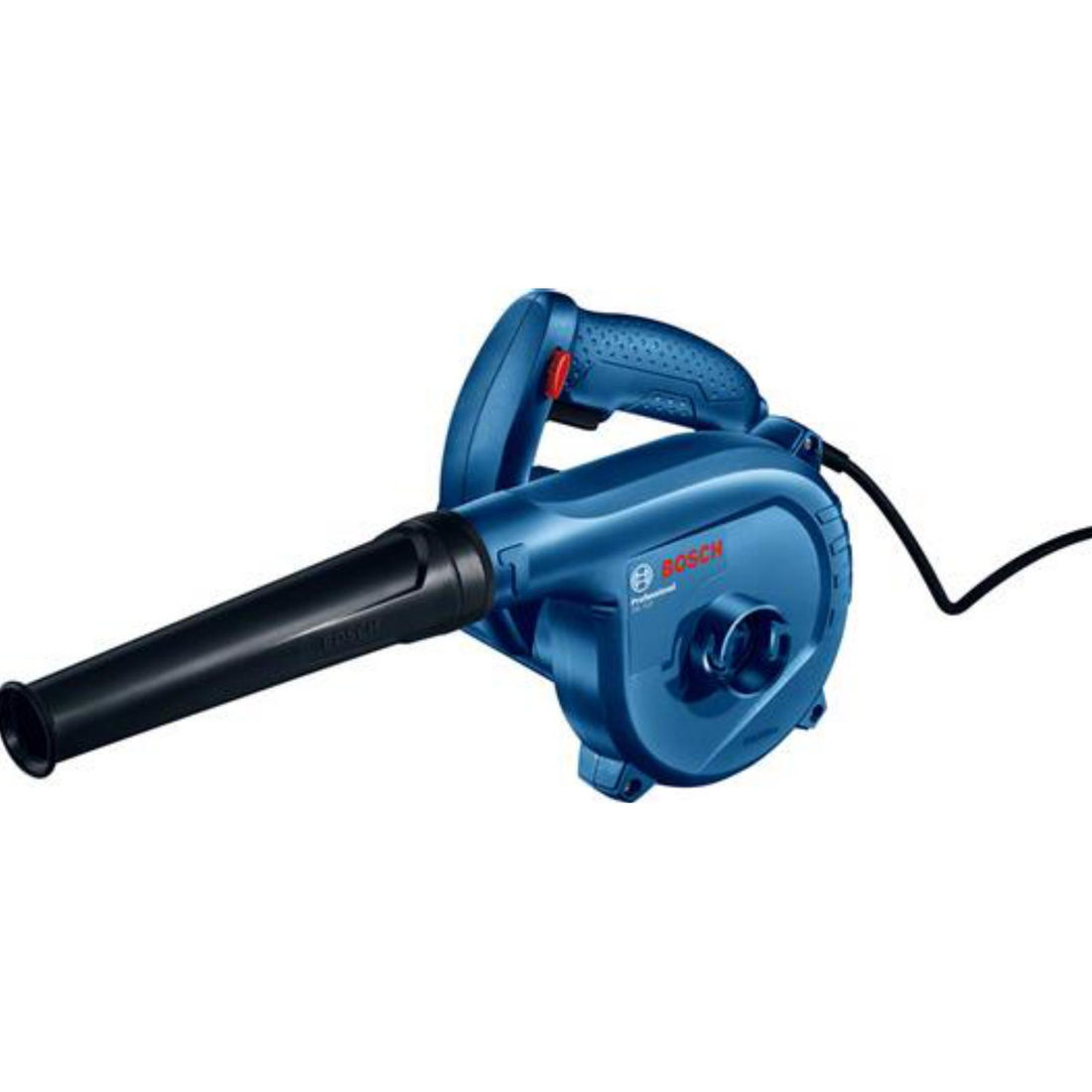top of page
GBL 620 Professional
Blower#### ProductIntro1#### The A-rated noise level of the power tool is typically as follows: Sound pressure level dB(A); Sound power level dB(A). Uncertainty K= dB.

 * Highlighted Data The A-rated noise level of the power tool is typically as follows: Sound pressure level dB(A); Sound power level dB(A). Uncertainty K= dB.
 * Highlighted Data The A-rated noise level of the power tool is typically as follows: Sound pressure level dB(A); Sound power level dB(A). Uncertainty K= dB.
TECHNICAL DATA
 Rated input power* Weight* Volumetric flow rate* No-load speed
 620 W 1.7 kg 3.5 m³/min 16,000 rpm
 Rated input power* Weight* Volumetric flow rate* No-load speed
 620 W 1.7 kg 3.5 m³/min 16,000 rpm